### Home > PC > Chapter 9 > Lesson 9.1.1 > Problem9-10

9-10.
1. A car which runs very slowly in the morning travels at a speed of 2t ft/s in the first t seconds after it starts for 0 ≤ t ≤ 50. Homework Help ✎

1. Draw the velocity versus time graph.

2. Use your graph to find the distance the car travels in the first t seconds after it starts.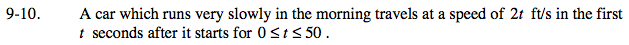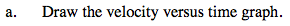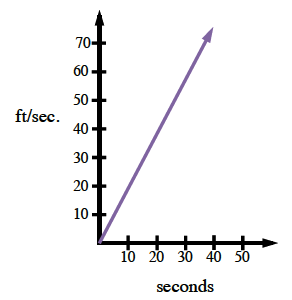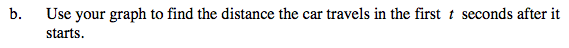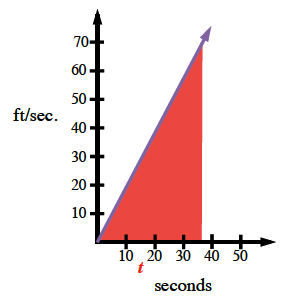$\text{Area}_\text{triangle}=\frac {\text{(base)(height)}}{2}$

Distance is the area under a velocity curve.

$\text{distance}=\frac{(t)(f(t))}{2}$

$\text{distance}=\frac{(t)(2t)}{2} = \frac{2t^{2}}{2} = t^2$